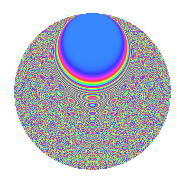# Properties

 Label 804.2.bfLevel 804 Weight 2 Character orbit bf Rep. character $$\chi_{804}(7,\cdot)$$ Character field $$\Q(\zeta_{66})$$ Dimension 1360 Newforms 2 Sturm bound 272 Trace bound 3

# Related objects

## Defining parameters

 Level: $$N$$ = $$804 = 2^{2} \cdot 3 \cdot 67$$ Weight: $$k$$ = $$2$$ Character orbit: $$[\chi]$$ = 804.bf (of order $$66$$ and degree $$20$$) Character conductor: $$\operatorname{cond}(\chi)$$ = $$268$$ Character field: $$\Q(\zeta_{66})$$ Newforms: $$2$$ Sturm bound: $$272$$ Trace bound: $$3$$ Distinguishing $$T_p$$: $$7$$

## Dimensions

The following table gives the dimensions of various subspaces of $$M_{2}(804, [\chi])$$.

Total New Old
Modular forms 2800 1360 1440
Cusp forms 2640 1360 1280
Eisenstein series 160 0 160

## Trace form

 $$1360q + 4q^{4} - 66q^{8} - 136q^{9} + O(q^{10})$$ $$1360q + 4q^{4} - 66q^{8} - 136q^{9} + 54q^{10} - 12q^{13} - 20q^{14} + 4q^{16} + 48q^{20} + 8q^{21} + 66q^{22} - 12q^{24} + 136q^{25} + 20q^{26} - 16q^{28} + 16q^{29} + 24q^{30} + 80q^{32} - 18q^{36} - 24q^{37} + 18q^{38} + 74q^{40} + 36q^{44} - 26q^{46} + 92q^{49} + 48q^{50} + 28q^{56} - 132q^{57} - 24q^{60} - 12q^{61} + 68q^{62} - 98q^{64} + 416q^{68} - 144q^{73} + 42q^{74} - 28q^{76} + 8q^{77} + 18q^{78} - 30q^{80} - 136q^{81} - 168q^{82} + 8q^{84} - 166q^{86} + 28q^{88} + 54q^{90} - 20q^{92} + 4q^{93} - 36q^{97} + 102q^{98} + O(q^{100})$$

## Decomposition of $$S_{2}^{\mathrm{new}}(804, [\chi])$$ into irreducible Hecke orbits

Label Dim. $$A$$ Field CM Traces $q$-expansion
$$a_2$$ $$a_3$$ $$a_5$$ $$a_7$$
804.2.bf.a $$680$$ $$6.420$$ None $$0$$ $$-68$$ $$0$$ $$4$$
804.2.bf.b $$680$$ $$6.420$$ None $$0$$ $$68$$ $$0$$ $$-4$$

## Decomposition of $$S_{2}^{\mathrm{old}}(804, [\chi])$$ into lower level spaces

$$S_{2}^{\mathrm{old}}(804, [\chi]) \cong$$ $$S_{2}^{\mathrm{new}}(268, [\chi])$$$$^{\oplus 2}$$